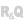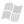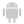HelpEditorsControls (window/page/report)ControlsTable Column controlProgramming automatic calculations in the columns of a Table controlWhen are the calculations performed?Retrieving the calculation resultModifying the characteristics of calculations through programmingExporting the results of calculationsSee alsoCalculations in the columns of a Table controlCharacteristics of columns found in a Table control in a page (prefix syntax)Characteristics of the columns in a Table control in a windowCharacteristics of the columns in a Table control in a window (prefix syntax)Characteristics of the columns of a Table control in a pageColumn of type AttributeConfiguring the titles of columns (Table control)Container column of a Table control (prefix syntax)Container column in a Table controlDefining columns of Table controls programmaticallyEvents associated with the columns (Table control)Programming automatic calculations in the columns of a Table controlProgramming automatic calculations in the columns of a Table control (prefix syntax)Programming the table cellsProperties associated with columns of Table controls
• When are the calculations performed?
• Retrieving the calculation result
• Modifying the characteristics of calculations through programming
• Properties that can be used on the calculation cells of columns
• Exporting the results of calculations
• Export via the AAF (Automatic Application Features) of Table control
• Export via the WLanguage functions
WINDEVWEBDEVWINDEV MobileOthersProgramming automatic calculations in the columns of a Table control
When are the calculations performed?
The calculations are performed:
• when initializing the Table control.
• when a process performs an addition or modification in a Table control with calculations:
• the totals are re-calculated at the end of process (or when using Multitask).
• if the value of a calculation is requested (see next paragraph), the calculation is instantly rerun.
TotalsEnabled is used to forbid or force the calculations performed in a Table control.
Caution: If the DisplayEnabled property is used on the Table control and is set to False, the automatic calculations are not updated.
Retrieving the calculation result
The result of calculations performed in a Table control can be handled through programming.
Several constants are used to identify the calculation row of a column:
 rowAverage Row containing the result of a mean. rowCount Row containing the result of the count. rowMaximum Row containing the maximum value of column cells. rowMinimum Row containing the minimum value of column cells. rowTotal Row containing the result of a total.

To retrieve the value of a calculation, use the following syntax:
<Column name>[<Type of calculation>]

Example:
// Retrieve the result for the average calculated in the COL_GRADE column
Modifying the characteristics of calculations through programming
WINDEV allows you to modify the characteristics (font, color, ...) of a column result through programming with several WLanguage properties. The WLanguage properties can be used to modify the color of result, its background color, its font, ... However, the content of result cell cannot be modified through programming.
To use a WLanguage property on the result of a column, the following syntax must be used:
<Column name>[<Type of calculation>].<Property>

Example:
// Color the mean result in red. The background is colored in blue

Properties that can be used on the calculation cells of columns

 BackgroundColor Used to find out and modify the background color of a calculation cell. Caption Used to find out and modify the caption of the row containing a calculation cell. Color Used to find out and modify the color of the text displayed in a calculation cell. Font Allows you to find out and modify the font used in a calculation cell. FontBold Used to find out and modify the "Bold" attribute for the column elements. FontCondensed Used to find out whether the characters of column elements are condensed or not, and to condense (or not) the characters of column elements. FontExtended Used to find out whether the characters of column elements are extended or not, and to extend (or not) the characters of column elements. FontItalic Used to find out and modify the "Italic" attribute for the column elements. FontLarge Used to find out whether the characters of column elements are enlarged or not, and to enlarge (or not) the characters of column elements. FontName Used to find out and modify the font used for the column elements. FontSize Used to find out and modify the size of the font used for the column elements. FontStrikeOut Used to find out and modify the "StrikeOut" attribute for a calculation cell. FontUnderlined Used to find out and modify the "Underline" attribute for the column elements. Height Used to find out and modify the height of a calculation cell. Name Used to find out the name of a calculation cell. Note Used to find out and modify the notes associated with a calculation cell. State Gets and changes the display state of the row containing the calculation cell. Visible Used to find out whether a column is visible and to make a column visible/invisible. Width Used to find out and modify the width of a calculation cell.
Exporting the results of calculationsExport via the AAF (Automatic Application Features) of Table control

The context menu of Table and TreeView Table controls contains several options to export the control content.
During this export, the results of automatic calculations are exported.
Remarks:
• Only the value is exported, not the caption.
• During the export in Excel, the cell corresponding to the calculation contains the corresponding Excel formula.

Export via the WLanguage functions

Several WLanguage functions can be used to export the content of a Table control in a specific format (Word, Excel, XML, ...). During this export, the rows corresponding to calculations are also exported by default.
To avoid exporting the calculation rows, all you have to do is specify the taNoTotal constant in the following functions:
 TableToClipboard Copies the content of a Table or TreeView Table control to the clipboard. TableToExcel Creates an Excel file with the data from a Table or TreeView Table control. TableToText Creates a character string from the data found in a Table or TreeView Table control. TableToWord Creates a Word file (.RTF) from the data found in a Table or TreeView Table control. TableToXML Creates an XML file from the data found in a Table or TreeView Table control.
Minimum version required
• Version 9